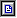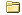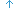We closed this forum 18 June 2010. It has served us well since 2005 as the ALPHA forum did before it from 2002 to 2005. New discussions are ongoing at the new URL http://forum.processing.org. You'll need to sign up and get a new user account. We're sorry about that inconvenience, but we think it's better in the long run. The content on this forum will remain online.Pages: 1loadStrings (Read 2700 times)
 AAK loadStrings Jun 18th, 2010, 11:13am   I'm trying to get a bunch of different files (292) into my program, but I keep getting the error "cannot convert from String[] to String." The pictures load fine, but I tried doing the same thing with the text files, and it doesn't work. What's different about strings that won't allow this to work? Code:```int numPics = 292; // change with number of picsint numPts = 866; // number of points in spectraPImage[] pics = new PImage[numPics];String[] spec = new String[numPics];void setup() {  size(1000,950);  smooth();  for ( int k = 0; k < numPics; k++) {    String imageName =  "S5_stamp_" + nf(k, 5) + ".png";    pics[k] = loadImage(imageName);    String spectra = "S5_hi_spectra_" + nf(k, 5) + ".txt";    spec[k] = loadStrings(spectra);      } ```TfGuy44 Re: loadStrings Reply #1 - Jun 18th, 2010, 11:25am   What's different is that loadStrings() returns an array of Strings, while loadImage() only returns a single PImage. You should make spec an array of arrays of Strings - a String [][] - since that's what you're really trying to use it as.AAK Re: loadStrings Reply #2 - Jun 18th, 2010, 12:13pm   That gives me the same error, if i try: Code:```int numPics = 292; // change with number of points, numPics = # picturesint numPts = 866; // number of points in spectraPImage[] pics = new PImage[numPics];String[][] spec;void setup() {  size(1000,950);  smooth();  for (int k = 0; k < numPics; k++) {    for (int h = 0; h < numPts; h++) {    String imageName =  "S5_stamp_" + nf(k, 5) + ".png";    pics[k] = loadImage(imageName);    String spectra = "S5_hi_spectra_" + nf(k, 5) + ".txt";    spec[k][h] = loadStrings(spectra);    }  } ```If I just try: Code:```int numPics = 292; // change with number of points, numPics = # picturesint numPts = 866; // number of points in spectraPImage[] pics = new PImage[numPics];String[][] spec;void setup() {  size(1000,950);  smooth();  for (int k = 0; k < numPics; k++) {    for (int h = 0; h < numPts; h++) {    String imageName =  "S5_stamp_" + nf(k, 5) + ".png";    pics[k] = loadImage(imageName);    String spectra = "S5_hi_spectra_" + nf(k, 5) + ".txt";    spec = loadStrings(spectra);    }  } ```I get "cannot convert from String[] to String[][]". It does actually work if I make spec just a String[] and don't specify spec[k], but that isn't what I want it to do. I want each spec[k] to be one filename so that I can then split the data from each file into the form I need for my graph.Pages: 1